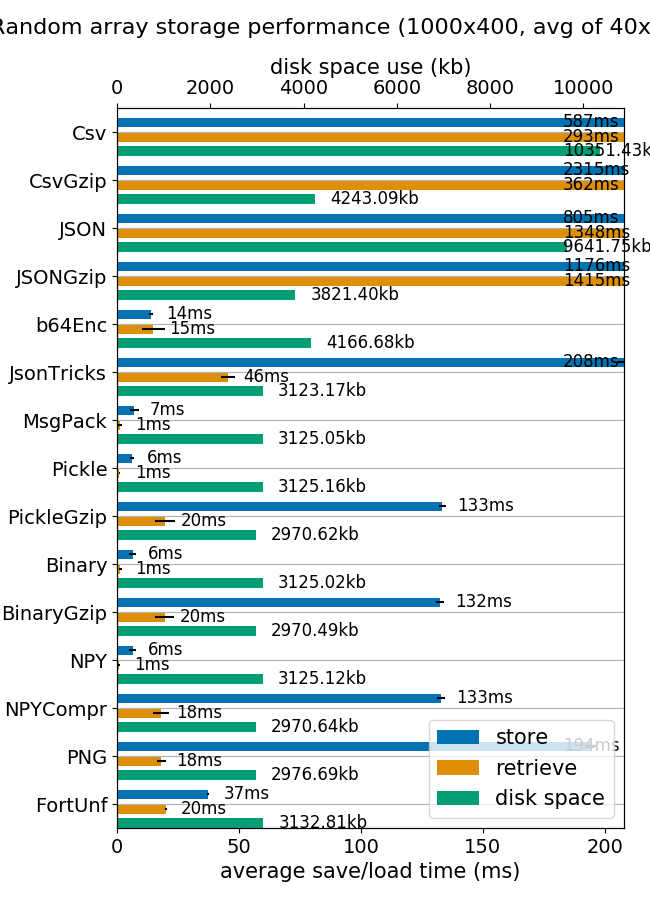# Python insert numpy array into sqlite3 database

ID : 131400

viewed : 8

Tags : pythonnumpysqlitepython

### Top 5 Answer for Python insert numpy array into sqlite3 database91

You could register a new `array` data type with `sqlite3`:

``import sqlite3 import numpy as np import io  def adapt_array(arr):     """     http://stackoverflow.com/a/31312102/190597 (SoulNibbler)     """     out = io.BytesIO()     np.save(out, arr)     out.seek(0)     return sqlite3.Binary(out.read())  def convert_array(text):     out = io.BytesIO(text)     out.seek(0)     return np.load(out)   # Converts np.array to TEXT when inserting sqlite3.register_adapter(np.ndarray, adapt_array)  # Converts TEXT to np.array when selecting sqlite3.register_converter("array", convert_array)  x = np.arange(12).reshape(2,6)  con = sqlite3.connect(":memory:", detect_types=sqlite3.PARSE_DECLTYPES) cur = con.cursor() cur.execute("create table test (arr array)") ``

With this setup, you can simply insert the NumPy array with no change in syntax:

``cur.execute("insert into test (arr) values (?)", (x, )) ``

And retrieve the array directly from sqlite as a NumPy array:

``cur.execute("select arr from test") data = cur.fetchone()  print(data) # [[ 0  1  2  3  4  5] #  [ 6  7  8  9 10 11]] print(type(data)) # <type 'numpy.ndarray'> ``82

I think that `matlab` format is a really convenient way to store and retrieve numpy arrays. Is really fast and the disk and memory footprint is quite the same.(image from mverleg benchmarks)

But if for any reason you need to store the numpy arrays into SQLite I suggest to add some compression capabilities.

The extra lines from unutbu code is pretty simple

``compressor = 'zlib'  # zlib, bz2  def adapt_array(arr):     """     http://stackoverflow.com/a/31312102/190597 (SoulNibbler)     """     # zlib uses similar disk size that Matlab v5 .mat files     # bz2 compress 4 times zlib, but storing process is 20 times slower.     out = io.BytesIO()     np.save(out, arr)     out.seek(0)     return sqlite3.Binary(out.read().encode(compressor))  # zlib, bz2  def convert_array(text):     out = io.BytesIO(text)     out.seek(0)     out = io.BytesIO(out.read().decode(compressor))     return np.load(out) ``

The results testing with MNIST database gives were:

``\$ ./test_MNIST.py :  99% remain: 0 secs    Storing 70000 images in 379.9 secs Retrieve 6990 images in 9.5 secs \$ ls -lh example.db  -rw-r--r-- 1 agp agp 69M sep 22 07:27 example.db \$ ls -lh mnist-original.mat  -rw-r--r-- 1 agp agp 53M sep 20 17:59 mnist-original.mat ``` ``

using `zlib`, and

``\$ ./test_MNIST.py :  99% remain: 12 secs    Storing 70000 images in 8536.2 secs Retrieve 6990 images in 37.4 secs \$ ls -lh example.db  -rw-r--r-- 1 agp agp 19M sep 22 03:33 example.db \$ ls -lh mnist-original.mat  -rw-r--r-- 1 agp agp 53M sep 20 17:59 mnist-original.mat ``

using `bz2`

Comparing `Matlab V5` format with `bz2` on SQLite, the bz2 compression is around 2.8, but the access time is quite long comparing to Matlab format (almost instantaneously vs more than 30 secs). Maybe is worthy only for really huge databases where the learning process is much time consuming than access time or where the database footprint is needed to be as small as possible.

Finally note that `bipz/zlib` ratio is around 3.7 and `zlib/matlab` requires 30% more space.

The full code if you want to play yourself is:

``import sqlite3 import numpy as np import io  compressor = 'zlib'  # zlib, bz2  def adapt_array(arr):     """     http://stackoverflow.com/a/31312102/190597 (SoulNibbler)     """     # zlib uses similar disk size that Matlab v5 .mat files     # bz2 compress 4 times zlib, but storing process is 20 times slower.     out = io.BytesIO()     np.save(out, arr)     out.seek(0)     return sqlite3.Binary(out.read().encode(compressor))  # zlib, bz2  def convert_array(text):     out = io.BytesIO(text)     out.seek(0)     out = io.BytesIO(out.read().decode(compressor))     return np.load(out)  sqlite3.register_adapter(np.ndarray, adapt_array) sqlite3.register_converter("array", convert_array)  dbname = 'example.db' def test_save_sqlite_arrays():     "Load MNIST database (70000 samples) and store in a compressed SQLite db"     os.path.exists(dbname) and os.unlink(dbname)     con = sqlite3.connect(dbname, detect_types=sqlite3.PARSE_DECLTYPES)     cur = con.cursor()     cur.execute("create table test (idx integer primary key, X array, y integer );")      mnist = fetch_mldata('MNIST original')      X, y =  mnist.data, mnist.target     m = X.shape     t0 = time.time()     for i, x in enumerate(X):         cur.execute("insert into test (idx, X, y) values (?,?,?)",                     (i, y, int(y[i])))         if not i % 100 and i > 0:             elapsed = time.time() - t0             remain = float(m - i) / i * elapsed             print "\r[%5d]: %3d%% remain: %d secs" % (i, 100 * i / m, remain),             sys.stdout.flush()      con.commit()     con.close()     elapsed = time.time() - t0     print     print "Storing %d images in %0.1f secs" % (m, elapsed)  def test_load_sqlite_arrays():     "Query MNIST SQLite database and load some samples"     con = sqlite3.connect(dbname, detect_types=sqlite3.PARSE_DECLTYPES)     cur = con.cursor()      # select all images labeled as '2'     t0 = time.time()     cur.execute('select idx, X, y from test where y = 2')     data = cur.fetchall()     elapsed = time.time() - t0     print "Retrieve %d images in %0.1f secs" % (len(data), elapsed)   if __name__ == '__main__':     test_save_sqlite_arrays()     test_load_sqlite_arrays() ``80

This works for me:

``import sqlite3 as sql import numpy as np import json con = sql.connect('test.db',isolation_level=None) cur = con.cursor() cur.execute("DROP TABLE FOOBAR") cur.execute("CREATE TABLE foobar (id INTEGER PRIMARY KEY, array BLOB)") cur.execute("INSERT INTO foobar VALUES (?,?)", (None, json.dumps(np.arange(0,500,0.5).tolist()))) con.commit() cur.execute("SELECT * FROM FOOBAR") data = cur.fetchall() print data data = cur.fetchall() my_list = json.loads(data) ``66

Happy Leap Second has it close but I kept getting an automatic casting to string. Also if you check out this other post: a fun debate on using buffer or Binary to push non text data into sqlite you see that the documented approach is to avoid the buffer all together and use this chunk of code.

``def adapt_array(arr):     out = io.BytesIO()     np.save(out, arr)     out.seek(0)     return sqlite3.Binary(out.read()) ``

I haven't heavily tested this in python 3, but it seems to work in python 2.757

The other methods specified didn't work for me. And well there seems to be a `numpy.tobytes` method now and a `numpy.fromstring` (which works on byte strings) but is deprecated and the recommended method is `numpy.frombuffer`.

``import sqlite3 import numpy as np  sqlite3.register_adapter(np.array, lambda arr: arr.tobytes())     sqlite3.register_converter("array", np.frombuffer) ``

I've tested it in my application and it works well for me on `Python 3.7.3` and `numpy 1.16.2`

`numpy.fromstring` gives the same outputs along with `DeprecationWarning: The binary mode of fromstring is deprecated, as it behaves surprisingly on unicode inputs. Use frombuffer instead`